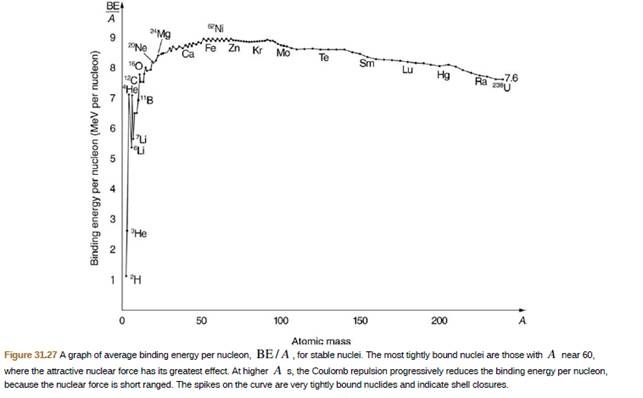Chapter 31, Problem 70PE

Chapter
Section
Textbook Problem

56Feis among the most tightly bound of all nuclides. It is more than 90% of natural iron. Note that 56Fe has even numbers of both protons and neutrons. Calculate BE/A, the binding energy per nucleon, for 56Fe and compare it with the approximate value obtained from the graph in Figure 31.27.To determine

The binding energy per nucleon of F56e .

Explanation

Given info:

From periodic table,

The atomic mass A of F56e is 55.9349375 u .

Number of protons Z present in F56e is 26 .

The number of neutron present in N

F56e is N=(AZ)=30 .

Formula used:

Formula to find the B.E. per nucleon is,

B.E.A=Z m p+N m nmtotalc2A

Equation-1

Here, mp is the mass of proton, mn is the mass of neutron, mtotal is the total mass and c is the speed of light.

Calculation:

To find the Binding energy per nucleon of F56esubstitute all the known values in equation-1

B.E.AF56e=26× 1

Still sussing out bartleby?

Check out a sample textbook solution.

See a sample solution

The Solution to Your Study Problems

Bartleby provides explanations to thousands of textbook problems written by our experts, many with advanced degrees!

Get Started

Explain the acronyms mks, SI, and cgs.

An Introduction to Physical Science

What are sister chromatids?

Human Heredity: Principles and Issues (MindTap Course List)

How does a covalent bond form?

Biology: The Dynamic Science (MindTap Course List)